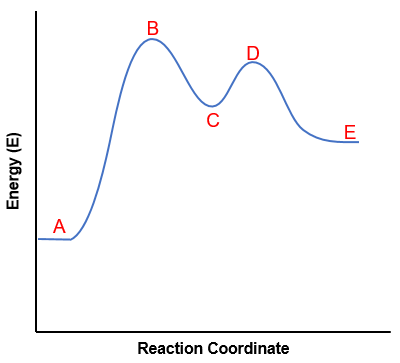# Problem: The activation energy for the reaction associated with the figure shown isa. B minus Ab. B minus Cc. D minus Ed. D minus Ce. D minus A

###### FREE Expert Solution

We’re being asked to identify the activation energy for the reaction associated with the figure below.Recall that an energy diagram is usually read from left to right

The components of a two-step energy diagram are:

79% (245 ratings)###### Problem Details

The activation energy for the reaction associated with the figure shown isa. B minus A
b. B minus C
c. D minus E
d. D minus C
e. D minus A